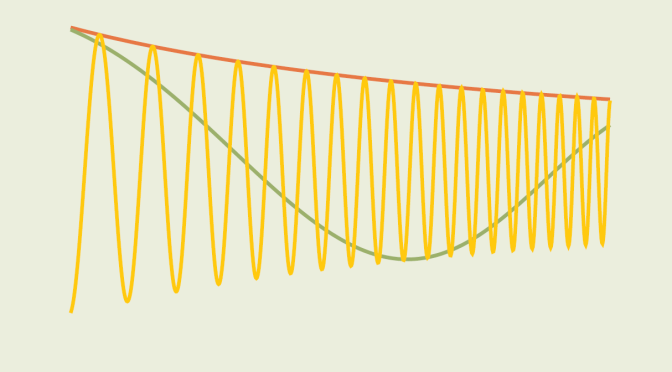# Differentiable functions converging to zero whose derivatives diverge (part1)

In this article, I consider real valued functions $$f$$ defined on $$(0,+\infty)$$ that converge to zero, i.e.:
$\lim\limits_{x \to +\infty} f(x) = 0$ If $$f$$ is differentiable what can be the behavior of its derivative as $$x$$ approaches $$+\infty$$?

Let’s consider a first example:
$\begin{array}{l|rcl} f_1 : & (0,+\infty) & \longrightarrow & \mathbb{R} \\ & x & \longmapsto & \frac{1}{x} \end{array}$ $$f_1$$ derivative is $$f_1^\prime(x)=-\frac{1}{x^2}$$ and we also have $$\lim\limits_{x \to +\infty} f_1^\prime(x) = 0$$. Let’s consider more sophisticated cases!

## A map converging to $$0$$ whose derivative has no limit but is bounded

For our second example, consider the map:
$\begin{array}{l|rcl} f_2 : & (0,+\infty) & \longrightarrow & \mathbb{R} \\ & x & \longmapsto & \frac{\sin(x^2)}{x} \end{array}$ As the $$\sin$$ function is bounded, the limit of $$f_2$$ as $$x$$ approaches $$+\infty$$ is $$0$$. What about its derivative $$\displaystyle f_2^\prime(x) = 2 \cos(x^2) – \frac{\sin (x^2)}{x^2}$$? Consider the sequence $$u_n=\sqrt{n \pi}$$ for $$n \ge 1$$. The value of $$f_2^\prime$$ at $$u_n$$ is $$2(-1)^n$$. As $$\lim\limits_{n \to +\infty} u_n = +\infty$$ we can conclude that $$f_2^\prime(x)$$ has no limit at $$+\infty$$. However for $$x \ge 1$$ we have:
$\vert f_2^\prime(x) \vert \le 2 \vert \cos(x^2) \vert + \left\vert \frac{\sin(x^2)}{x^2} \right\vert \le 3$ so $$f_2^\prime$$ is bounded in the neighborhood of $$+\infty$$.

## A map converging to $$0$$ whose derivative has no limit and is unbounded

For our last example, we consider the function:
$\begin{array}{l|rcl} f_3 : & (0,+\infty) & \longrightarrow & \mathbb{R} \\ & x & \longmapsto & \frac{\sin(x^3)}{x} \end{array}$ Again the limit of $$f_3$$ as $$x$$ approaches $$+\infty$$ is also $$0$$. $$f_3$$ derivative is $$\displaystyle f_3^\prime(x) = 3x \cos(x^3) – \frac{\sin (x^3)}{x^2}$$.

Consider the sequences:
$a_n=\sqrt{2 n \pi} \text{ and } b_n=\sqrt{(2n+1) \pi}$ For $$n \ge 1$$ we have:
$f_3^\prime(a_n)=3 a_n \text{ and } f_3^\prime(b_n)=-3 b_n$ The limit of both $$(a_n)$$ and $$(b_n)$$ as $$n$$ approaches $$+\infty$$ is $$\infty$$. Therefore $$f_3^\prime$$ has no limit and is unbounded in the neighborhood of $$+\infty$$.

None of the functions $$f_2$$ and $$f_3$$ is monotonic. I will come back later on with an example of a decreasing map converging to zero but whose derivative is unbounded in the neighborhood of $$+\infty$$.